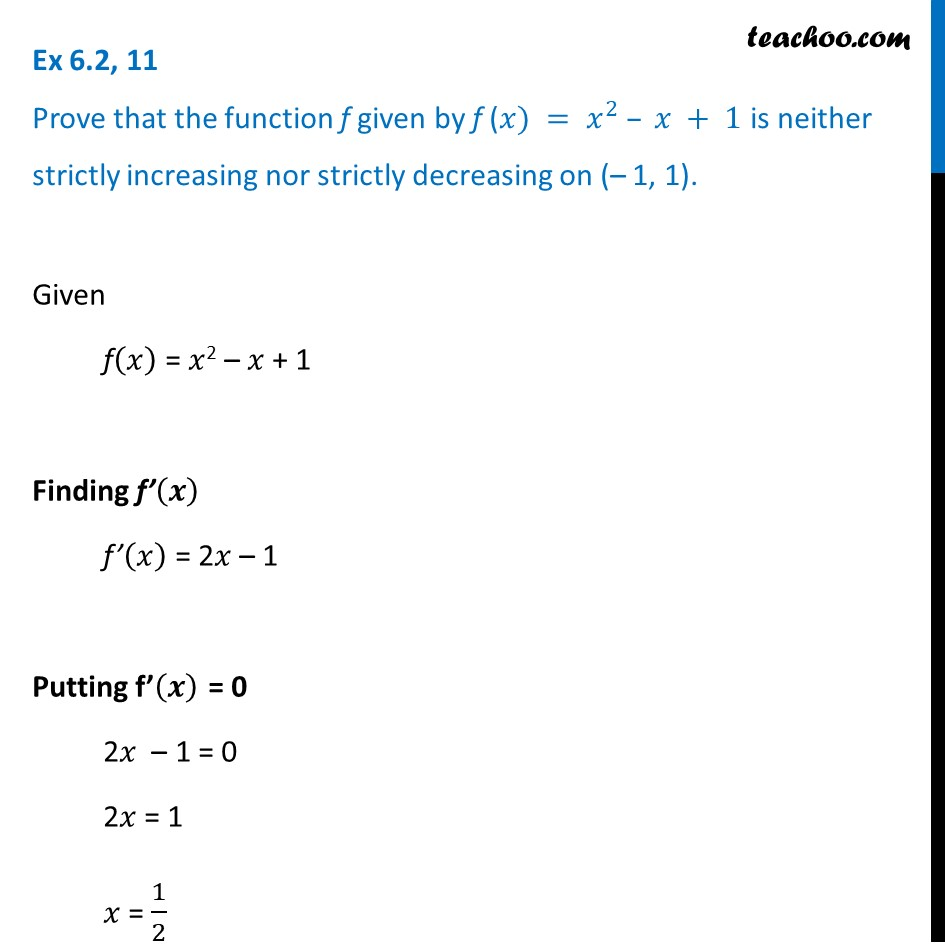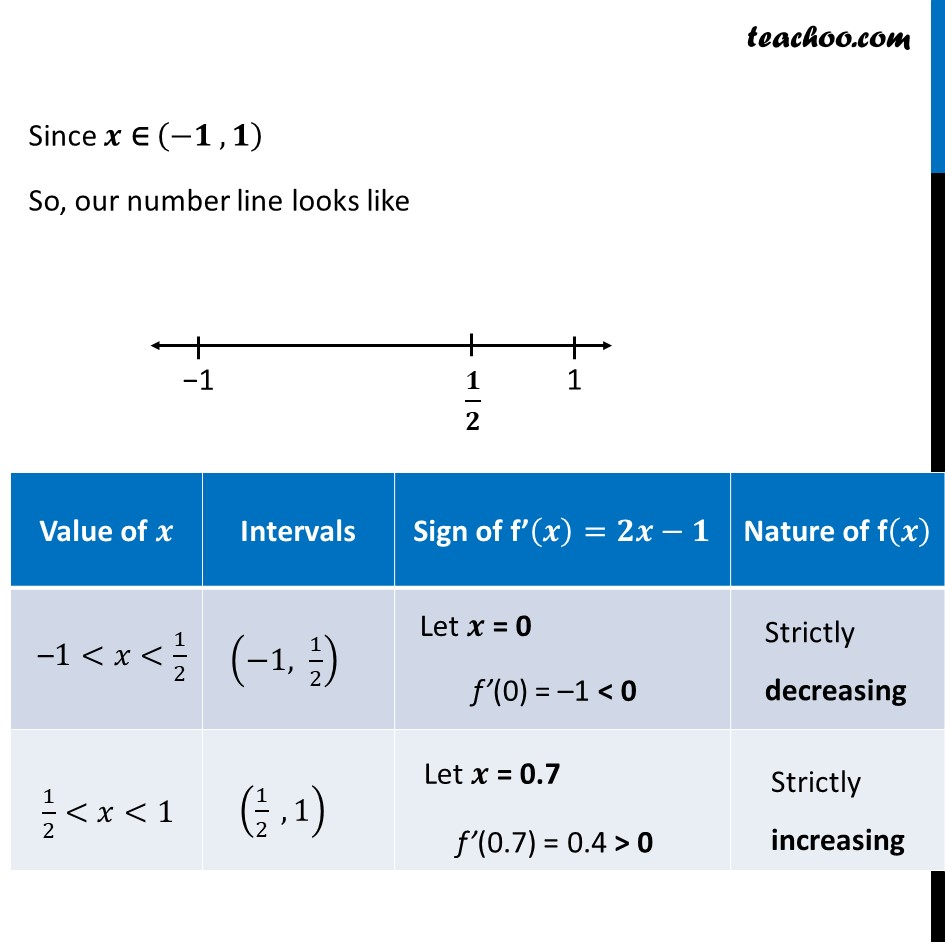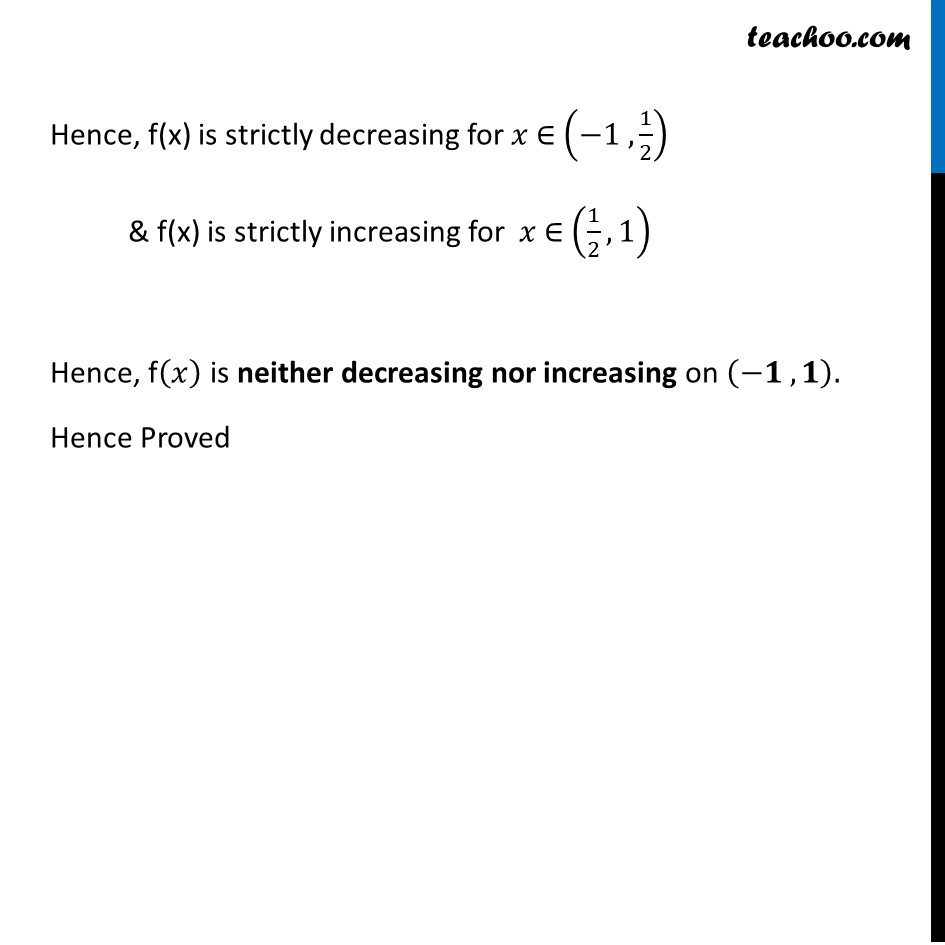Ex 6.2

Chapter 6 Class 12 Application of Derivatives
Serial order wiseLearn in your speed, with individual attention - Teachoo Maths 1-on-1 Class

### Transcript

Ex 6.2, 11 Prove that the function f given by f (𝑥) = 𝑥^2 – 𝑥 + 1 is neither strictly increasing nor strictly decreasing on (– 1, 1).Given f(𝑥) = 𝑥2 – 𝑥 + 1 Finding f’(𝒙) f’(𝑥) = 2𝑥 – 1 Putting f’(𝒙) = 0 2𝑥 – 1 = 0 2𝑥 = 1 𝑥 = 1/2 Since 𝒙 ∈ (−𝟏 , 𝟏) So, our number line looks like Hence, f(x) is strictly decreasing for 𝑥 ∈ (−1 , 1/2) & f(x) is strictly increasing for 𝑥 ∈ (1/2, 1) Hence, f(𝑥) is neither decreasing nor increasing on (−𝟏 , 𝟏). Hence Proved